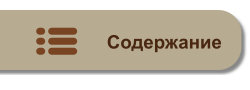MyTetra Share Делитесь знаниями!Range
Время создания: 01.10.2017 15:06
Раздел: Go
Запись: xintrea/mytetra_db_mcold/master/base/1506859571ayy2tloec8/text.html на raw.githubusercontent.com

The range keyword is used in for loop to iterate over items of an array, slice, channel or map. With array and slices, it returns the index of the item as integer. With maps, it returns the key of the next key-value pair. Range either returns one value or two. If only one value is used on the left of a range expression, it is the 1st value in the following table.

 Range expression 1st Value 2nd Value(Optional) Array or slice a [n]E index i int a[i] E String s string type index i int rune int map m map[K]V key k K value m[k] V channel c chan E element e E none

Example

The following paragraph shows how to use range −

`package main`

`import "fmt"`

`func main() {`
`   /* create a slice */`
`   numbers := []int{0,1,2,3,4,5,6,7,8} `
`   `
`   /* print the numbers */`
`   for i:= range numbers {`
`      fmt.Println("Slice item",i,"is",numbers[i])`
`   }`
`   `
`   /* create a map*/`
`   countryCapitalMap := map[string] string {"France":"Paris","Italy":"Rome","Japan":"Tokyo"}`
`   `
`   /* print map using keys*/`
`   for country := range countryCapitalMap {`
`      fmt.Println("Capital of",country,"is",countryCapitalMap[country])`
`   }`
`   `
`   /* print map using key-value*/`
`   for country,capital := range countryCapitalMap {`
`      fmt.Println("Capital of",country,"is",capital)`
`   }`
`}`

When the above code is compiled and executed, it produces the following result −

`Slice item 0 is 0`
`Slice item 1 is 1`
`Slice item 2 is 2`
`Slice item 3 is 3`
`Slice item 4 is 4`
`Slice item 5 is 5`
`Slice item 6 is 6`
`Slice item 7 is 7`
`Slice item 8 is 8`
`Capital of France is Paris`
`Capital of Italy is Rome`
`Capital of Japan is Tokyo`
`Capital of France is Paris`
`Capital of Italy is Rome`
`Capital of Japan is Tokyo`MyTetra Share v.0.58